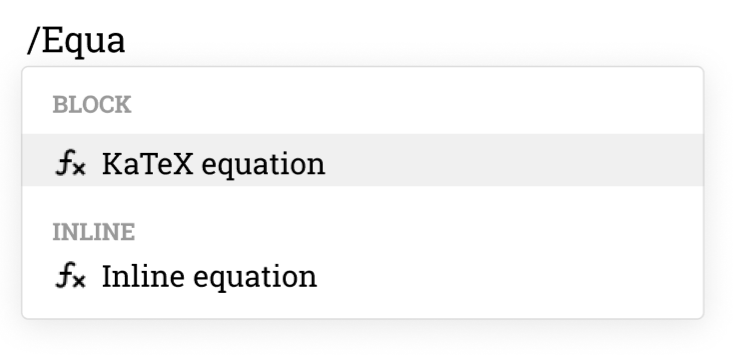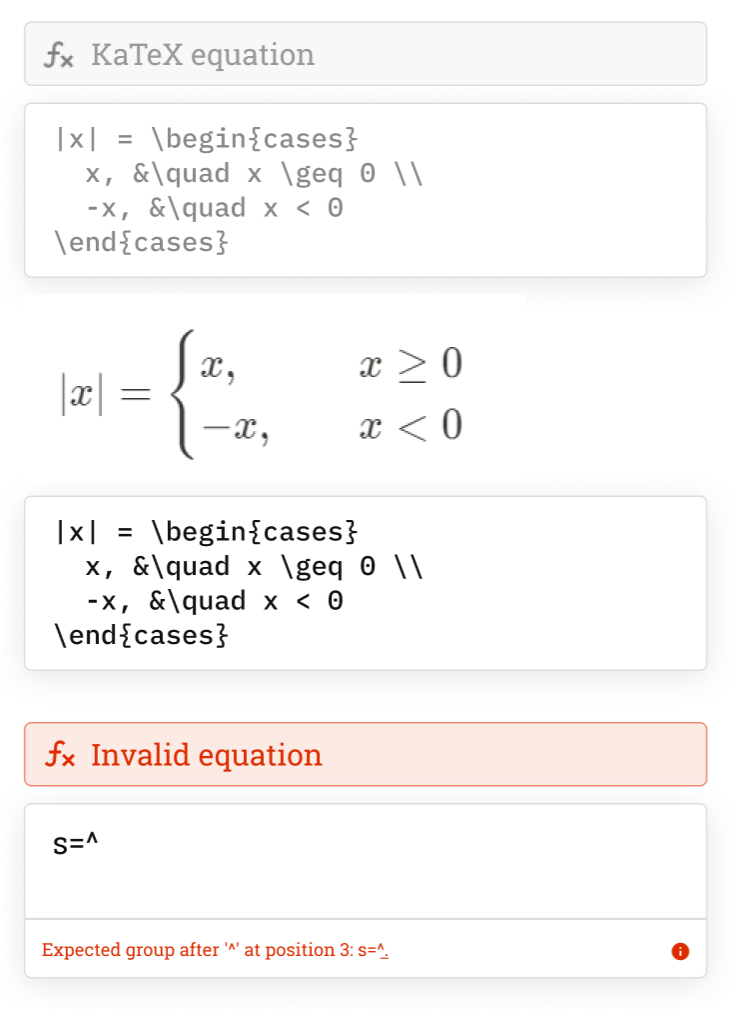# Colabra & LaTeX

Insert math equations into your electronic lab notebook.

Colabra's LaTeX feature allows researchers to easily input inline and block equations within their electronic lab notebook experimental notes.

This feature is especially useful for scientists and researchers working in fields such as physics, chemistry, and mathematics as it allows them to easily write and format mathematical equations, formulas, and expressions in a precise and unambiguous way.

The KaTeX block makes it easier for researchers to write and format equations in a consistent and professional manner, regardless of their level of technical expertise.

## Features

### Inline equations

Insert short math equations inline.How to use Type /Inline equation, enter your KaTeX equation, and press Enter.

### Block equations

Insert complex KaTeX equations in your experimental notes.How to use Type /KaTeX equation, enter your KaTeX code, and press Enter.